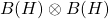# Real rank ofVon | Juni 20, 2020

Given a separable, infinite-dimensional Hilbert space, what is the real rank of the minimal tensor product?

Background/Motivation: The real rank is a noncommutative dimension theory that was introduced by Brown and Pedersen in ​​. It associates to each C*-algebraa number (its real rank). The lowest and most interesting value is zero. One can think of C*-algebras of real rank zero as zero-dimensional, noncommutative spaces. (There are other noncommutative dimension theories, and they lead to different concepts of zero- or low-dimensional noncommutative spaces.) For topological spacesand, the product theorem for covering dimension shows that under certain weak assumptions (for example, both spaces are metric, or compact) we have. One might therefore expect that, but it was shown in ​​ that this does not hold: there exists C*-algebrasandsuch thatLater, Osaka showed in ​​ that one can even take. Indeed, it is well known that von Neumann algebras have real rank zero, and hence so does. Osaka showed thatdoes not have real rank zero. This raises the question of what the real rank ofis, see Question 3.3 in .

1. 
L.G. Brown, G.K. Pedersen, C*-algebras of real rank zero, Journal of Functional Analysis. 99 (1991) 131–149. https://doi.org/10.1016/0022-1236(91)90056-b.
2. 
K. Kodaka, H. Osaka, Real Rank of Tensor Products of C*-Algebras, Proceedings of the American Mathematical Society. 123 (1995) 2213–2215. https://doi.org/10.2307/2160959.
3. 
H. Osaka, Certain C*-algebras with non-zero real rank and extremal richness, MATH. SCAND. 85 (1999) 79. https://doi.org/10.7146/math.scand.a-13886.